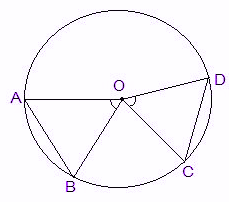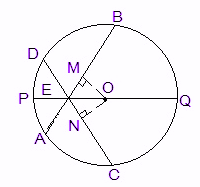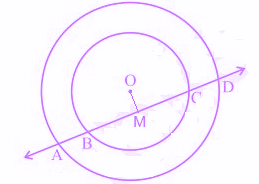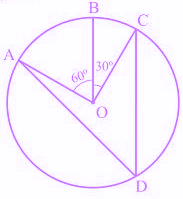# MCQ Questions for Class 9 Maths Chapter 10 Circles with Answers

## MCQ Questions for Class 9 Maths Chapter 10 Circles with Answers

MCQs from Class 9 Maths Chapter 10 – Circles are provided here to help students prepare for their upcoming Maths exam.

MCQs from CBSE Class 9 Maths Chapter 10: Circles

1) The center of the circle lies in______ of the circle.
a. Interior
b. Exterior
c. Circumference
d. None of the above

2) The longest chord of the circle is:
b. Arc
c. Diameter
d. Segment

3) Equal _____ of the congruent circles subtend equal angles at the centers.
a. Segments
c. Arcs
d. Chords

Explanation: See the figure below:Let ΔAOB and ΔCOD are two triangles inside the circle.

OA = OC and OB = OD (radii of the circle)

AB = CD (Given)

So, ΔAOB ≅ ΔCOD (SSS congruency)

∴ By CPCT rule, ∠AOB = ∠COD.

Hence, this prove the statement.

4) If chords AB and CD of congruent circles subtend equal angles at their centres, then:
a. AB = CD
b. AB > CD
d. None of the above

Explanation: Take the reference of the figure from above question.

In triangles AOB and COD,

∠AOB = ∠COD (given)

OA = OC and OB = OD (radii of the circle)

So, ΔAOB ≅ ΔCOD. (SAS congruency)

∴ AB = CD (By CPCT)

5) If there are two separate circles drawn apart from each other, then the maximum number of common points they have:
a. 0
b. 1
c. 2
d. 3

6) The angle subtended by the diameter of a semi-circle is:
a. 90
b. 45
c. 180
d. 60

Explanation: The semicircle is half of the circle, hence the diameter of the semicircle will be a straight line subtending 180 degrees.

7) If AB and CD are two chords of a circle intersecting at point E, as per the given figure. Then:a.∠BEQ > ∠CEQ
b. ∠BEQ = ∠CEQ
c. ∠BEQ < ∠CEQ
d. None of the above

Explanation:

OM = ON (Equal chords are always equidistant from the centre)

OE = OE (Common)

∠OME = ∠ONE (perpendiculars)

So, ΔOEM ≅ ΔOEN (by RHS similarity criterion)

Hence, ∠MEO = ∠NEO (by CPCT rule)

∴ ∠BEQ = ∠CEQ

8) If a line intersects two concentric circles with centre O at A, B, C and D, then:
a. AB = CD
b. AB > CD
c. AB < CD
d. None of the above

Explanation: See the figure below:From the above fig., OM ⊥ AD.

Therefore, AM = MD — 1

Also, since OM ⊥ BC, OM bisects BC.

Therefore, BM = MC — 2

From equation 1 and equation 2.

AM – BM = MD – MC

∴ AB = CD

9) In the below figure, the value of ∠ADC is:a. 60°
b. 30°
c. 45°
d. 55°

Explanation: ∠AOC = ∠AOB + ∠BOC

So, ∠AOC = 60° + 30°

∴ ∠AOC = 90°

An angle subtended by an arc at the centre of the circle is twice the angle subtended by that arc at any point on the rest part of the circle.

So,

= 1/2 × 90° = 45°

10) In the given figure, find angle OPR.a. 20°
b. 15°
c. 12°
d. 10°

Explanation: The angle subtended by an arc at the centre of the circle is twice the angle subtended by that arc at any point on the circle.

So, ∠POR = 2 × ∠PQR

We know the values of angle PQR as 100°

So, ∠POR = 2 × 100° = 200°

∴ ∠POR = 360° – 200° = 160°

Now, in ΔOPR,

OP and OR are the radii of the circle

So, OP = OR

Also, ∠OPR = ∠ORP

By angle sum property of triangle, we knwo:

∠POR + ∠OPR + ∠ORP = 180°

∠OPR + ∠OPR = 180° – 160°

As, ∠OPR = ∠ORP

2∠OPR = 20°

Thus, ∠OPR = 10°